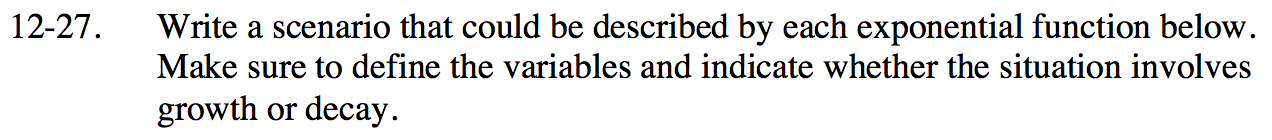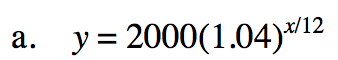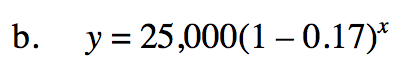### Home > INT2 > Chapter 12 > Lesson 12.1.2 > Problem12-27

12-27.
1. Write a scenario that could be described by each exponential function below. Make sure to define the variables and indicate whether the situation involves growth or decay. Homework Help ✎

1. y = 2000(1.04)x/12

2. y = 25,000(1 – 0.17)xAnswers vary, but one possible scenario could be: The amount of money, y, in an account after x years if the starting balance is \$2000 and the monthly interest is 4% exponential growth.